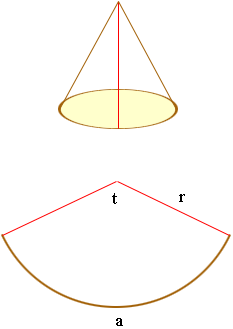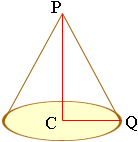Quandaries and Queries Hello I am trying to build crayfish traps; one of the components is a cone shaped entry section. The cone I want to make would be 12” in diameter at the base and 12” in height, from base to peak. I need a formula to calculate the dimensions and a method of transferring the shape onto a flat piece of material. Once I have made a model from heavy paper to confirmed the shape is what I want I will make a metal mesh screen version of the same thing. This will become the entry cone to my Crayfish trap. Thanks for your help. Steve Hi Steve, If you have a cone and cut it, by a line perpendicular to the circular base, and lay it out flat, you will have a sector of a circle. You can construct a cone by reversing this process.In the diagram above I have labeled the radius of the sector as r inches, the length of the arc as a inches and the angle at the center as t radians. I want to measure the angle in radians since this gives a nice relationship among the three variables a = rt Thus, if you know two of these three numbers you can calculate the third. The length of the arc a is the circumference of the base of the cone. Your cone has a radius of 6 inches at the base so a = 26 = 12inches. If you draw a line from the apex P of the cone to the center C of the base and then a line from the center of the base to a point Q on the bounding circle you form a right triangle PCQ.|PC| = 12 and |CD| = 6 and by Pythagoras' Theorem |PQ|2 = |PC|2 + |CQ|2 = 144 + 36 = 180 Thus |PQ| = 13.42 inches. From the first diagram r = |PQ| and hence the radius of the sector that forms the cone is 13.42 inches. Thus t = a/r = 12/13.42 = 0.894radians. To transform the angle to degrees you need to multiply by 180/0.894180/= 160.9 degrees On your heavy paper draw an arc with a radius of 13.4 inches and measure an angle of 160.9 degrees to form the sector. Cut it out and roll it up to form a cone and it will be the dimensions you want. I actually did this a couple of years ago to make a much smaller trap than you are building. You might want to make the angle a little larger than 160.9 degrees so that the edges of the mesh overlap a little to allow you to fasten them together. Making the model from paper first is a good idea. Good luck with his, Harley Go to Math Central